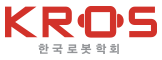[ Article ]
The Journal of Korea Robotics Society - Vol. 16, No. 2, pp.147-154
ISSN: 1975-6291 (Print) 2287-3961 (Online)
Print publication date 01 Jun 2021
Received 12 Jan 2021 Revised 25 Feb 2021 Accepted 26 Feb 2021

# Fast and Safe Contact Establishment Strategy for Biped Walking Robot

1Student, Graduate School of Convergence Science and Technology, Seoul National University, Seoul, Korea hslee1989@snu.ac.krjjs916@snu.ac.krjune992@snu.ac.kr
이족 보행 로봇을 위한 빠르고 안전한 접촉 생성 전략

Correspondence to: Professor, Corresponding author: Graduate School of Convergence Science and Technology, Seoul National University, Seoul, Korea and Manager, Advanced Institutes of Convergence Technology, Suwon, Korea ( park73@snu.ac.kr)

## Abstract

One of the most challenging issues when robots interact with the environment is to establish contact quickly and avoid high impact force at the same time. The proposed method implements the passive suspension system using the redundancy of the torque-controlled robot. Instead of utilizing the actual mechanical compliance, the distal joints near the end-effector are controlled to act as a virtual spring-damper system with low feedback gains. The proximal joints are precisely controlled to push the mid-link, which is defined as the boundary link between the proximal and distal joints, towards the environment with high feedback gains. Compared to the active compliance methods, the contact force measurements or estimates are not required for contact establishment and the control time delay problems do not occur correspondingly. The proposed method was applied to the landing foot control of the 12-DoF biped robot DYROS-RED in the simulations. In the results, the impact force during landing was significantly reduced at the same collision speed.

## Keywords:

Contact Establishment, Impact Force, Torque-Controlled Robot, Landing Foot Control

## 1. Introduction

Interaction with the environment is essential for robots to be used in daily life. One of the most challenging issues in establishing contact is to move the end-effector quickly and avoid high impact forces at the same time. The impact force is produced by both physical impulse and the feedback controller. Moving the end-effector quickly and precisely requires high feedback gains in the presence of modeling errors, which leads to larger contact forces in the time period between physical impact and the controller’s recognition of the contact event.

Several approaches have been developed to handle contact tasks by implementing active compliance for rigid manipulators. The typical ways are impedance control and hybrid position/force control using force/torque (F/T) sensors at the wrist. The contact can be perceived using thresholds of the F/T sensor measurements. Similarly, the landing foot of the humanoid robot was controlled using the F/T sensor at the ankle during walking[4,5]. Some methods used a momentum-based disturbance observer or tactile sensors instead of the F/T sensor.

Active compliance, however, typically has time delay problems because of the noise in the contact force measurements or estimates. On the contrary, passive mechanical compliance can react to the contact event with no control time delay. For example, torsional springs and dampers were located in every joint of a manipulator for peg-in-hole tasks. Since passive compliance has a limitation in precise control, a method was developed to implement both active and passive compliance using a robotic arm with a compliant wrist. It can reduce physical impact force by increasing contact duration while tracking performance is less reduced compared to the fully passive mechanical system.

Our approach for fast and safe contact establishment is inspired by human behavior, as shown in [Fig. 1]. To press a button with a fingertip, all finger joints are kept still at the beginning of the motion and a hand moves forward to push the fingertip towards the button ([Fig. 1(a)]). When the contact occurs, the configuration of distal joints near the fingertip is deformed, acting as a mechanical suspension system. This can reduce physical impact force by increasing collision time. Similarly, the swing leg absorbs impact force while the torso moves downwards during landing ([Fig. 1(b)]). We have modeled the distal joints as a passive spring-damper system, which is pushed by the proximal joints towards the environment.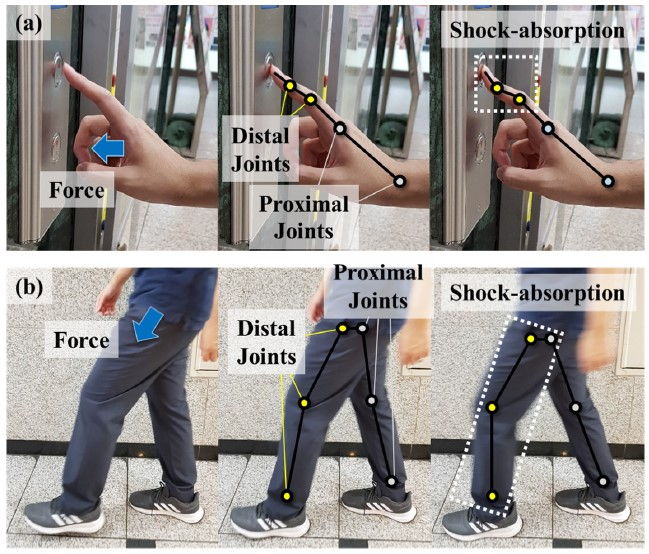Snapshots of human motion to (a) press a button with a fingertip. (b) land on the ground

In this paper, a novel method to establish contact quickly and safely is proposed for redundant torque-controlled robots. The redundancy is resolved to implement a passive spring-damper system near the contact point using the distal joints. The suspension links are pushed towards the environment by controlling the proximal joints with high feedback gains. Compared to active compliance methods, the proposed method has no control time delay problem and does not require the contact force measurements. The proximal joints are controlled with high feedback gains to improve the tracking performance. Unlike the manipulator with a compliant wrist, our method can be applied to any redundant robot which does not have the actual mechanical compliance.

In Sec. II, the concept of the proposed method is introduced and the torque solution is derived for both joint-space and task-space control. The proposed method is applied to the landing foot control of a biped robot and its simulation results are presented in Sec. III. Conclusions are given in Sec. IV.

## 2. Contact Establishment Strategy

### 2.1 Concept of the Proposed Method

[Fig. 2] shows the concept of the proposed method implemented by a 4 degrees-of-freedom (DoF) planar manipulator. The goal is to establish contact between the end-effector and the object quickly with small impact force. Each number of the proximal and distal joints is 2. The mid-link is defined as the boundary link between them ([Fig. 2(a)]).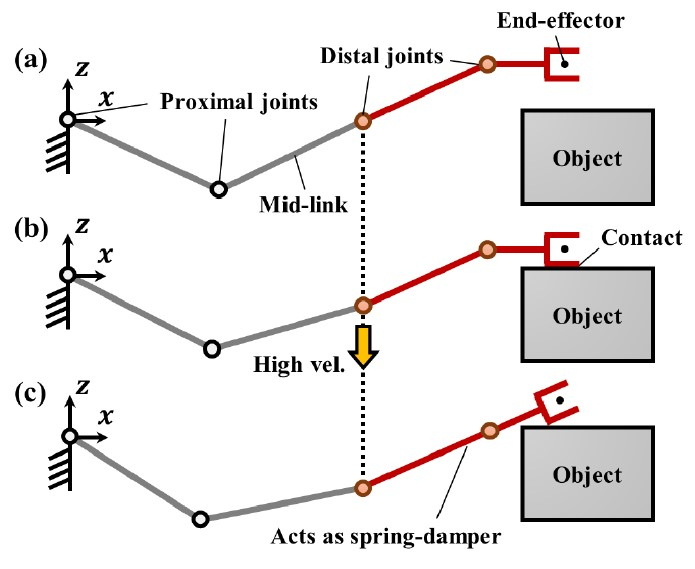Contact establishment using the proposed method for a 4-DoF planar manipulator. (a) Distal Joints are controlled to keep their initial values with low feedback gains. (b) Proximal joints are controlled to push the end-effector downwards with high feedback gains. (c) The distal joints acts as a passive spring-damper reducing impact force

In [Fig. 2(b)], the 2-DoF position of the mid-link is controlled with high feedback gains by the proximal joints. It pushes the end-effector towards the object for contact establishment. The distal joints are controlled to keep their initial posture with low feedback gains, implementing a passive spring-damper system. Their deformation can absorb 2-DoF impact force by increasing collision time, as shown in [Fig. 2(c)]. This concept can be applied to any torque-controlled robot with redundancy such as in [Fig. 3].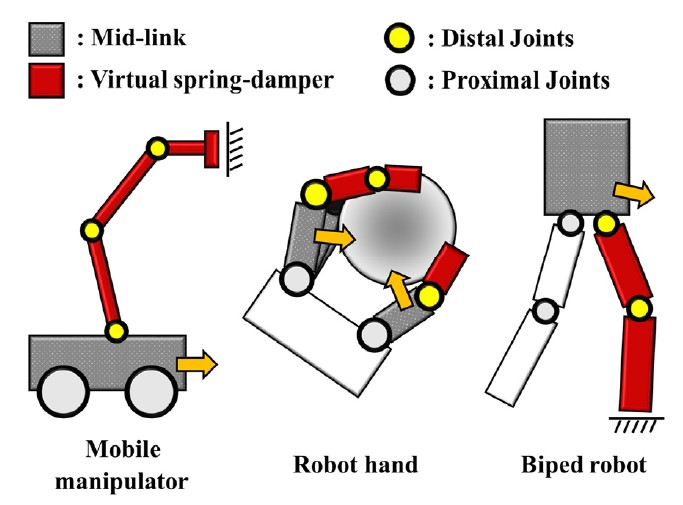Application of the proposed method to various types of redundant robots. The grey link indicates the mid-link and the red links act as a passive spring-damper system

When the torque solutions of the proximal joints are obtained, the effective mass of the suspension links has to be considered using the dynamics model. Otherwise, the tracking performance of the end-effector will be affected by the uncompensated dynamics especially when the number of the distal joints are large or the suspension links are heavy. In addition, the impact force can be absorbed only in the direction where the distal joints can move. When their configuration is near singularity, the stiffness of the virtual suspension becomes very large. Therefore, the initial posture of the distal joints has to be non-singular in the direction where the end-effector is pushed towards the object.

The proposed method can be implemented in both joint and task space. In the latter case, the end-effector is controlled to keep its relative position with respect to the mid-link and the passive compliance can be adjusted separately in each direction of the Cartesian coordinate.

### 2.2 Obtaining Torque Solution

The proposed method can be applied to either a fixed-base manipulator or a floating-base robot. In this paper, dynamics of a floating-base robot is derived, which can be a general expression of a fixed-base dynamics. Its derivation will be introduced briefly and more detailed descriptions can be found in the previous work.

We defined k and n=k+6 as the number of actuated and total joints, respectively. The number of ground-contact is given as c. Then, the joint-space dynamics is given by

 $\mathbit{A}\stackrel{¨}{\mathbit{q}}+\mathbit{b}+\mathbit{g}+{\mathbit{J}}_{\mathit{C}}^{\mathit{T}}{\mathbit{f}}_{\mathit{c}}={\left({\mathbf{S}}^{k}\right)}^{T}{\mathbit{\Gamma }}^{k}$ (1)

where A is the n×n mass matrix, b is the n×1 centrifugal/Coriolis vector, and g is the n×1 gravitational vector. Jc is the n×c Jacobian matrix for the ground-contact space and fc is the c×1 ground reaction force (GRF) vector. Γk is the k×1 torque vector of the actuated joints and Sk is the k×n selection matrix, given by [0k×6 Ik×k].

The GRF fc in Eqn. (1) does not include the contact force between the object and the end-effector, fee, which is considered as disturbance force in this paper. Using the kinematic constraints on the ground-contact, Eqn. (1) is projected into the task space, given by

 $\mathbit{\Lambda }\stackrel{¨}{\mathbit{x}}+\mathbit{\mu }+\mathbit{p}=\mathbit{F},$ (2)

where Λ is the t×t mass matrix, μ is the t×1 centrifugal/Coriolis vector, and p is the t×1 gravitational vector. t is the number of tasks. x and F are the t×1 vector of task position and force, respectively.

The relationship between the task force F and the torque solution Γk is given by

 $\mathbit{F}={\overline{\mathbit{J}}}^{T}{\left({\mathbf{S}}^{k}\right)}^{T}{\mathbit{\Gamma }}^{k},$ (3)

where J is the t×n Jacobian matrix for the task and $\overline{\mathbit{J}}$ is the dynamically-consistent inverse of J. Then, Γk can be obtained by solving Eqn. (3) analytically or numerically.

The controlled DoFs of the mid-link and the suspension are defined as t1 and t2, respectively, satisfying t=t1+t2. When the suspension is implemented in the joint space, the total task x is written as

 $\mathbit{x}={\left[{\mathbit{x}}_{\mathrm{m}\mathrm{i}\mathrm{d}}^{\mathrm{T}}{\mathbit{x}}_{\mathrm{j}}^{\mathrm{T}}\right]}^{\mathrm{T}},$ (4)

where xmid is the t1×1 position vector of the mid-link and xj is the t2×1 position vector of the distal joints. The corresponding Jacobian matrix is given by

 $\mathbit{J}={\left[{\mathbit{J}}_{\mathrm{m}\mathrm{i}\mathrm{d}}^{\mathrm{T}}{\mathbit{J}}_{\mathrm{j}}^{\mathrm{T}}\right]}^{\mathrm{T}},$ (5)

where Jmid is the t1×n Jacobian matrix for the mid-link position and Jj is the t2×n Jacobian matrix for the distal joint angles. Jj is simply given as a selection matrix ${\mathbf{S}}_{{\mathrm{t}}_{2}}=\left[{\mathbf{0}}_{{\mathrm{t}}_{2}×\left(\mathrm{n}-{\mathrm{t}}_{2}\right)}{\mathbf{I}}_{{\mathrm{t}}_{2}×{\mathrm{t}}_{2}}\right].$

The task force F is obtained using the proportional-derivative (PD) controller.

 $\mathbit{F}=\mathbit{\Lambda }\left\{{\mathbit{k}}_{\mathrm{p}}\left({\mathbit{x}}_{\mathrm{d}}-\mathbit{x}\right)-{\mathbit{k}}_{\mathrm{v}}\stackrel{˙}{\mathbit{x}}\right\}+\mathbit{\mu }+\mathbit{p},$ (6)

where kp and kv are the t×t diagonal matrix for proportional and derivative gains, respectively. xd is the t×1 vector of the desired task position, given by

 ${\mathbit{x}}_{\mathrm{d}}={\left[{\mathbit{x}}_{\mathrm{m}\mathrm{i}\mathrm{d}, \mathrm{d} }^{\mathrm{T}}{\mathbit{x}}_{\mathrm{j}, \mathrm{d}}^{\mathrm{T}}\right]}^{\mathrm{T}}$ (7)

xmid,d is the desired mid-link position to push the end-effector towards the object. xj,d is set to the initial value of xj at the beginning of the task.

When the suspension is implemented in the task space, the total task x changes from Eqn. (4) to

 (8)

where xee is the t2×1 position vector of the end-effector. The corresponding Jacobian matrix is given by

 (9)

where Jee is the t2×n Jacobian matrix for the end-effector position. The desired task position xd is given by

 ${\mathbit{x}}_{\mathrm{d}}={\left[{\mathbit{x}}_{\mathrm{m}\mathrm{i}\mathrm{d}, \mathrm{d}}^{\mathrm{T}}{}_{}{\mathbit{x}}_{\mathrm{e}\mathrm{e},\mathrm{d}}^{\mathrm{T}}\right]}^{\mathrm{T}},$ (10)

where xee, d is the desired end-effector position. In order to control the relative position of the end-effector with respect to the mid-link, xee, d is given by

 ${\mathbit{x}}_{\mathrm{e}\mathrm{e},\mathrm{d}}={\mathbit{x}}_{\mathrm{m}\mathrm{i}\mathrm{d}, {\mathrm{t}}_{2}}+\overline{\mathbit{x}}.$ (11)

xmid, t2 is the t2×1 position vector of the mid-link in the direction where the end-effector is controlled. $\overline{\mathbit{x}}$ is the vector of the initial distance between the mid-link and the end-effector at the beginning of the task, given by [xee-xmid, t2]init. The task force F is obtained from Eqn. (6). In this work, the PD gains were set high for the mid-link and low for the end-effector, or the suspension, through experiments. The impact force can be reduced significantly when the suspension gains are low. However, the mid-link may not be able to push the end-effector quickly because of the inertia of the suspension links. Therefore, it is recommended to set the suspension gains high until the end-effector is located near the goal position.

## 3. Application to Biped Walking

### 3.1 Landing Foot Control

The proposed method was applied to landing foot control of a 12-DoF biped robot as shown in [Fig. 4]. For fast walking, the foot has to land on the ground at a high speed with small impact force. During the single-support-phase (SSP), the 6-DoF suspension is implemented between the torso and the landing foot using task space control. The task space coordinate is given in [Fig. 4]. The stiffness of the suspension is set low in the z direction and high in the rest of the directions. As the mid-link, the torso is precisely controlled to push the landing foot towards the ground using high feedback gains. For position tracking, the feedback controller is constructed by Eqn. (6). The swing leg is not fully stretched during walking to avoid its singular configuration.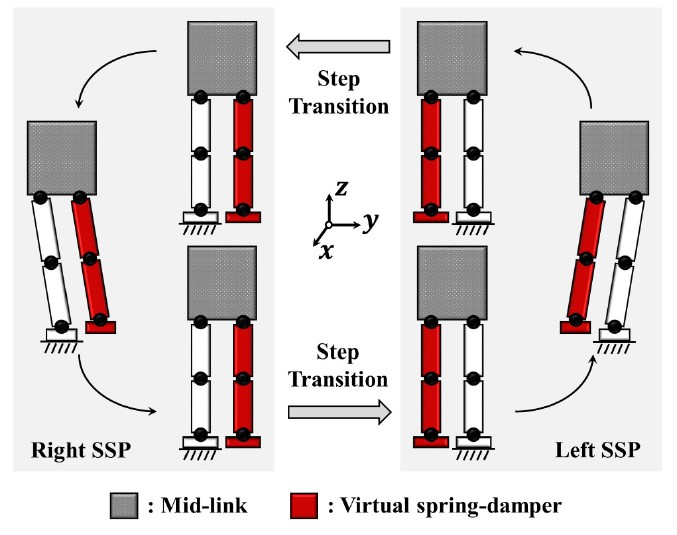Application of the proposed method to landing foot control of a biped robot. A virtual spring-damper is implemented between the torso and the landing foot in the z direction

The initial distance of the suspension, $\overline{x}$, is selected when the foot is lifted to the maximum height at the first step. From that moment, the z-motion of the landing foot is determined by the z-motion of the torso and the spring-damper gains. At the beginning of the next SSP, the landing foot changes to the next supporting foot and the height of the torso starts to be restored. The next swing foot is lifted by the torso until the length of the swing leg becomes x. Then, the torso is pushed downwards again and the rest of the procedure is the same as in the previous step.

Generally, the amount of time spent in the double-support-phase (DSP) decreases as the walking speed increases. In this work, the torque solution in the DSP was obtained by simply interpolating the torque solutions in the previous and next SSP under the assumption that the walking speed is sufficiently high.

### 3.2 Reactive Walking

In this work, a lateral walking motion is generated using the Reactive walking method. In order to improve reactivity to external disturbances, the walking motion is generated depending on the current state of the robot. The single SSP is divided into two phases, which are the single-support-deceleration-phase (SSDP) and single-support-acceleration-phase (SSAP) as shown in [Fig. 5].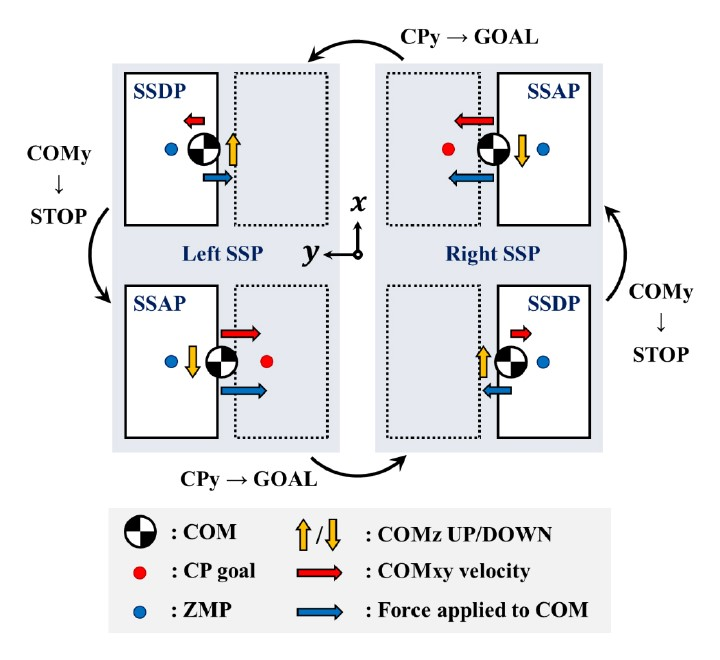Walking phases used in a reactive walking method. One SSP is divided into two phases, which are single-support-deceleration-phase (SSDP) and single-support-acceleration-phase (SSAP). We added a z-directional motion of the CoM to implement the proposed method

In the SSAP, the center-of-mass (CoM), or the torso, of the robot is accelerated towards the landing foot in the x and y direction until the current capture-point (CP) reaches its goal position. The force to push the CoM is determined by the zero-moment-point (ZMP) location. The detailed description of the CP and ZMP can be found in the previous works[13,14]. When the CP reaches its goal position, the swing foot is commanded to land on the ground and the walking phase proceeds to the SSDP in the next step. In the SSDP, the swing foot is lifted and the ZMP is located to push the CoM against the current direction of the movement until the CoM stops. Then, the walking phase changes to the SSAP and the same procedure is repeated.

The reactive walking method is based on the linear inverted pendulum model which requires constant height of the CoM. However, the CoM or the torso moves up and down during walking in the proposed method. Therefore, a general inverted pendulum model is used instead to calculate the desired force at the CoM for the given ZMP location in the y direction.

 ${\stackrel{¨}{x}}_{\mathrm{c}\mathrm{o}\mathrm{m},\mathrm{y}\mathrm{d}}=\frac{{\stackrel{¨}{x}}_{\mathrm{c}\mathrm{o}\mathrm{m}·\mathrm{z}}+g}{{x}_{\mathrm{c}\mathrm{o}\mathrm{m},\mathrm{z}}}\left({x}_{\mathrm{c}\mathrm{o}\mathrm{m},\mathrm{y}}-{p}_{\mathrm{y}}\right),$ (12)

where py is the given ZMP y-position and $\stackrel{¨}{x}$com,yd is the CoM y-acceleration to generate py. xcom,y and xcom,z are the CoM position in the y and z direction, respectively. g is the acceleration of gravity.

In summary, the torso movement is generated by the reactive walking method in the y-direction and by the proposed method in the z-direction. The remaining position and orientation of the torso is kept still during walking for simplicity.

### 3.3 Simulation Results

[Fig. 6] shows the model of the 12-DoF biped robot DYROS-RED ancion. To verify the performance of the proposed controller, three different gains of the spring-damper in the z-direction were selected. The lowest gain was 625 for kp and 50 for kp, the middle one was 2500-100, and the highest was 5625-150. Other gains for the remaining tasks were kept the same in all cases. The desired CoM z-acceleration was determined by experiments to match the collision velocities in all cases and the measurements of the impact force were compared. The time periods of the SSP and DSP were about 0.5 sec and 0.2 sec, respectively. The simulation environment was constructed using a dynamics-based simulator RoboticsLab and the control frequency was set to 1000 Hz.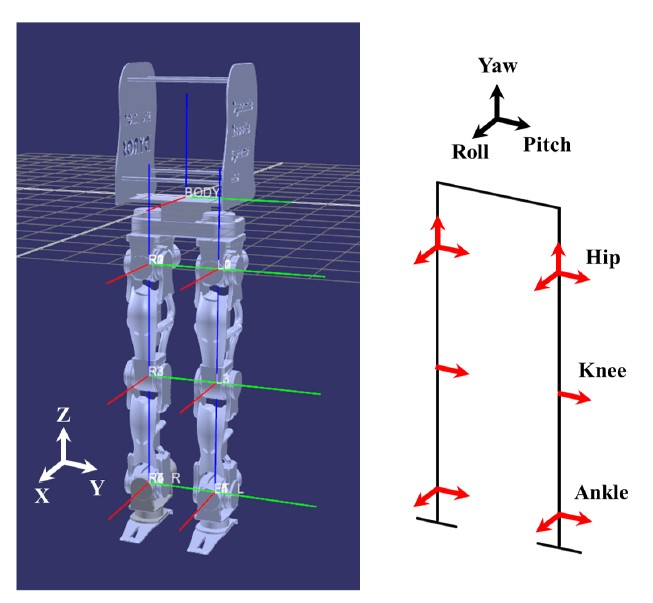A model of the 12-DoF biped robot DYROS-RED (left) and its joint configuration (right)

[Fig. 7] shows the trajectories of the CoM, ZMP, and landing foot for 2 cycles corresponding to the suspension gains. While the CoM and ZMP location in the y direction are almost the same in all cases, the step time becomes longer as the gain increases([Fig. 7(a)]). This will be explained later. When the first contact occurs around t=0.5 sec, the CoM movements in the z direction are significantly different depending on the suspension gains ([Fig. 7(b)]). The CoM keeps descending after the contact when the gains are low, increasing the collision time and thus reducing the physical impact force. When the gains are high, on the other hand, the CoM bounces up due to the short collision time and large impact force. This leads to the fluctuation of the landing foot z-position and the contact loss for a while correspondingly, as shown in [Fig. 7(c)].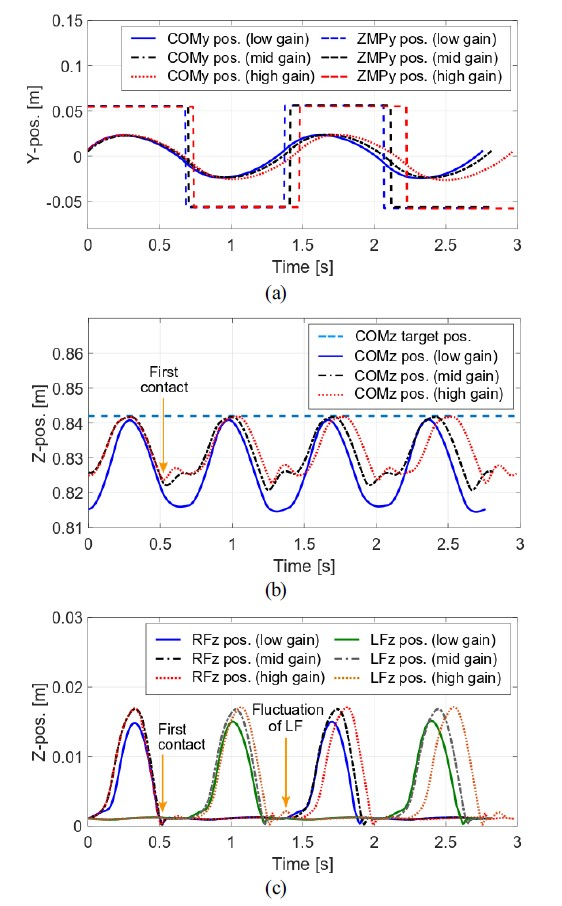Walking trajectories generated in the simulation for 2 cycles corresponding to the suspension gains. (a) The CoM and ZMP y-motion. (b) The CoM z-motion and its target position. (c) The landing foot z-motion

[Fig. 8(a)] shows the velocities of the CoM and the landing foot from the moment of contact during the right SSP. The collision velocities of the landing foot are almost the same as about -0.125 m/s for the two suspension gains. The reason why the step time becomes longer as the gain increases in [Fig. 7] is well illustrated in the CoM y-velocity. When the suspension gains are high, the CoM y-velocity remains nearly unchanged from about 0.06 sec to 0.09 sec because of the large impact force applied at around t=0.05 sec ([Fig. 8(b)]). Since the reactive walking controller uses the CP as a criterion for the step transition, the step time becomes longer due to the late arrival of the CP on its goal position. In other words, the proposed method can increase the reactive walking speed by reducing the impact force which disturbs the CoM movement.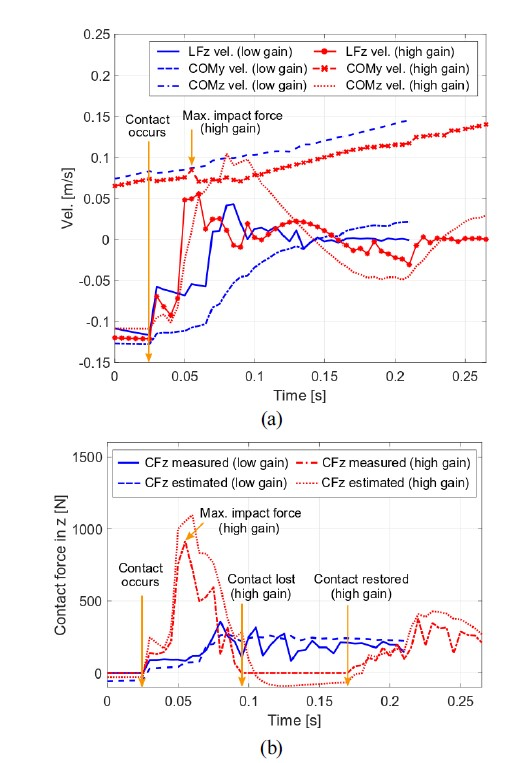The simulation results from the moment of contact during the right SSP. (a) The y and z-velocity of the CoM and the z-velocity of the landing foot. (b) The Contact force measured by the F/T sensor at the ankle and estimated by the spring-damper force of the swing leg

[Fig. 8(b)] shows the contact force measurements depending on the suspension gains. The contact is lost from around t=0.1 sec to 0.17 sec when the gains are high, as observed in the fluctuation of the landing foot z-position in [Fig. 7]. The maximum impact force is 915 N for the high gains and 356 N for the low gains, leading to 61% reduction at the same collision speed. In addition, the contact force can be estimated by the spring-damper force of the swing leg in the controller without the F/T measurements. The estimation results were similar to the F/T measurements as shown in [Fig. 8(b)]. The snapshots of the torso movement during landing are presented in [Fig. 9].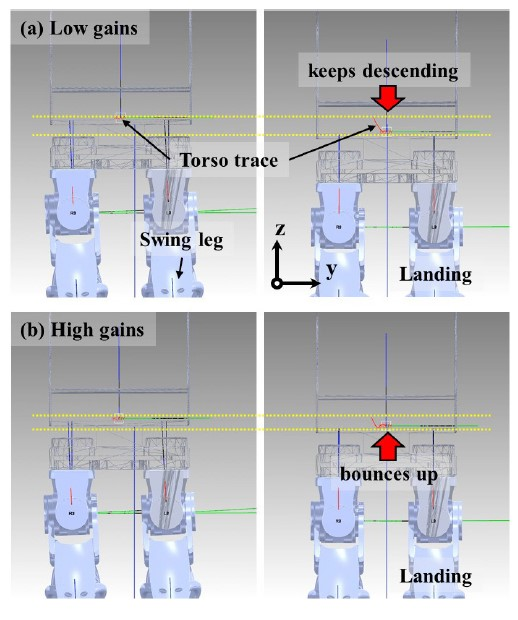Snapshots of the torso movement during landing. The torso (a) keeps descending after landing when the spring-damper gains are low. (b) bounces up after landing when the gains are high

## 4. Conclusions

The proposed method implements the passive suspension system using the redundancy of the torque-controlled robot without the actual mechanical compliance. Therefore, the F/T measurements are not required for contact establishment and the control time delay problems do not occur compared to the active compliance methods. When the contact occurs, the deformation of the suspension increases the collision time and thus reduces the physical impact force at the same collision velocity. The proposed method was applied to the landing foot control of the 12-DoF biped robot DYROS-RED. The simulation results showed that the impact force was significantly reduced by 61% at the same landing speed. In addition, the contact force was estimated by the spring-damper force of the swing leg in the controller without the F/T measurements. The proposed method can be applied to any torque-controlled robots with redundancy such as humanoid robots, quadruped robots, and mobile manipulators.

Theoretical backgrounds on finding spring-damper gains will be presented in future works. Besides, the performance of the proposed controller will be verified by the experiments using real robots.

## Acknowledgments

This work was supported by the Technology Innovation Program (No. 10060081) funded by the Ministry of Trade, Industry & Energy (MI, Korea) and by the National Research Foundation of Korea (NRF) grant funded by the Korea government (MSIT) (No. 2021R1A2C3005914)

## References

• N. Hogan, “Impedance Control: An Approach to Manipulation: Part I — Theory,” Journal of Dynamic Systems, Measurement, and Control, vol. 107, no. 1, pp. 1-7, 1985. [https://doi.org/10.1115/1.3140702]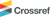• M. H. Raibert and J. J. Craig, “Hybrid Position/Force Control of Manipulators,” Journal of Dynamic Systems, Measurement, and Control, vol. 102, no. 2, pp. 126-133, 1981. [https://doi.org/10.1115/1.3139652]• G. S. Kanakis, F. Dimeas, G. A. Rovithakis, and Z. Doulgeri, “Prescribed contact establishment of a robot with a planar surface under position and stiffness uncertainties,” Robotics and Autonomous Systems, vol. 104, pp. 99-108, Jun., 2018. [https://doi.org/10.1016/j.robot.2018.03.005]• H. Wang, Y. F. Zheng, Y. Jun, and P. Oh, “DRC-hubo walking on rough terrains,” 2014 IEEE International Conference on Technologies for Practical Robot Applications (TePRA), Woburn, MA, USA, pp. 1-6, 2014. [https://doi.org/10.1109/TePRA.2014.6869151]• S. Kim, M. Kim, J. Lee, S. Hwang, J. Chae, B. Park, H. Cho, J. Sim, J. Jung, H. Lee, S. Shin, M. Kim, W. Choi, Y. Lee, S. Park, J. Oh, Y. Lee, S. Lee, M. Lee, S. Yi, K. K. C. Chang, N. Kwak, and J. Park, “Team snu’s control strategies for enhancing a robot’s capability: Lessons from the 2015 darpa robotics challenge finals,” Journal of Field Robotics, vol. 34, no. 2, pp. 359-380, 2017. [https://doi.org/10.1002/rob.21678]• E. Magrini, F. Flacco, and A. De Luca, “Control of generalized contact motion and force in physical human-robot interaction,” 2015 IEEE International Conference on Robotics and Automation (ICRA), Seattle, WA, USA, pp. 2298-2304, 2015. [https://doi.org/10.1109/ICRA.2015.7139504]• H. Zhang and N. N. Chen, “Control of contact via tactile sensing,” IEEE Transactions on Robotics and Automation, vol. 16, no. 5, pp. 482-495, Oct., 2000. [https://doi.org/10.1109/70.880799]• S.-K. Yun, “Compliant manipulation for peg-in-hole: Is passive compliance a key to learn contact motion?,” 2008 IEEE International Conference on Robotics and Automation, Pasadena, CA, USA, pp. 1647-1652, 2008. [https://doi.org/10.1109/ROBOT.2008.4543437]• N. Uyama, H. Nakanishi, K. Nagaoka, and K. Yoshida, “Impedance-based contact control of a free-flying space robot with a compliant wrist for non-cooperative satellite capture,” 2012 IEEE/RSJ International Conference on Intelligent Robots and Systems, Vilamoura-Algarve, Portugal, pp. 4477-4482, 2012. [https://doi.org/10.1109/IROS.2012.6386082]• J. Park and O. Khatib, “Contact consistent control framework for humanoid robots,” 2006 IEEE International Conference on Robotics and Automation, Orlando, FL, USA, pp. 1963-1969, 2006. [https://doi.org/10.1109/ROBOT.2006.1641993]• K. Mitobe, N. Mori, Y. Nasu, and N. Adachi, “Control of a biped walking robot during the double support phase,” Autonomous Robots, vol. 4, no. 3, pp. 287-296, 1997. [https://doi.org/10.1023/A:1008896010368]• Y. Lee and J. Park, “Reactive Bipedal Walking Method for Torque Controlled Robot,” 2018 IEEE International Conference on Robotics and Automation (ICRA), Brisbane, QLD, Australia, pp. 395-402, 2018. [https://doi.org/10.1109/ICRA.2018.8460668]• J. Pratt, J. Carff, S. Drakunov, and A. Goswami, “Capture point: A step toward humanoid push recovery,” 2006 6th IEEE-RAS International Conference on Humanoid Robots, Genova, Italy, pp. 200-207, 2006. [https://doi.org/10.1109/ICHR.2006.321385]• S. Kajita, F. Kanehiro, K. Kaneko, K. Fujiwara, K. Harada, K. Yokoi, and H. Hirukawa, “Biped walking pattern generation by using preview control of zero-moment point,” 2003 IEEE International Conference on Robotics and Automation, Taipei, Taiwan, pp. 1620-1626, 2003. [https://doi.org/10.1109/ROBOT.2003.1241826]이 호 상

2013 서울대학교 기계항공공학부(학사)

2013~현재 서울대학교 융합과학기술대학원 석박사 통합과정

관심분야: Torque-based Whole-body Control

정 재 석

2015 서울대학교 전기정보공학부(학사)

2015~현재 서울대학교 융합과학기술대학원 석박사 통합과정

관심분야: Torque-based Control Considering Joint Elasticity

안 준 휘

2016 성균관대학교 기계공학부(학사)

2016~현재 서울대학교 융합과학기술대학원 석박사 통합과정

관심분야: Multi-contact Control, Contact State Estimation

박 재 흥

1995 서울대학교 항공우주공학과(학사)

1999 서울대학교 항공우주공학과(석사)

2006 Stanford University Aero/Astro(박사)

2009~현재 서울대학교 융합과학기술대학원 교수

관심분야: Robot-environment Interaction, Contact Force Control, Multi-contact Control, Whole-body Dynamic Control, Bio-mechanics

### [Fig. 1]Snapshots of human motion to (a) press a button with a fingertip. (b) land on the ground

### [Fig. 2]Contact establishment using the proposed method for a 4-DoF planar manipulator. (a) Distal Joints are controlled to keep their initial values with low feedback gains. (b) Proximal joints are controlled to push the end-effector downwards with high feedback gains. (c) The distal joints acts as a passive spring-damper reducing impact force

### [Fig. 3]Application of the proposed method to various types of redundant robots. The grey link indicates the mid-link and the red links act as a passive spring-damper system

### [Fig. 4]Application of the proposed method to landing foot control of a biped robot. A virtual spring-damper is implemented between the torso and the landing foot in the z direction

### [Fig. 5]Walking phases used in a reactive walking method. One SSP is divided into two phases, which are single-support-deceleration-phase (SSDP) and single-support-acceleration-phase (SSAP). We added a z-directional motion of the CoM to implement the proposed method

### [Fig. 6]A model of the 12-DoF biped robot DYROS-RED (left) and its joint configuration (right)

### [Fig. 7]Walking trajectories generated in the simulation for 2 cycles corresponding to the suspension gains. (a) The CoM and ZMP y-motion. (b) The CoM z-motion and its target position. (c) The landing foot z-motion

### [Fig. 8]The simulation results from the moment of contact during the right SSP. (a) The y and z-velocity of the CoM and the z-velocity of the landing foot. (b) The Contact force measured by the F/T sensor at the ankle and estimated by the spring-damper force of the swing leg

### [Fig. 9]Snapshots of the torso movement during landing. The torso (a) keeps descending after landing when the spring-damper gains are low. (b) bounces up after landing when the gains are high